# Building Go binaries with Tensorflow: On packaging a Keras Model for serving

Let’s walk through a quick setup to define a workflow for serving a `keras` model packaged in a Go binary with TensorFlow backend engine. Keras is a high level Python API that makes it very easy to construct a deep learning model. It can seamlessly operate with TensorFlow as one of the supported ML engines. Typically, all such high level work is done in Python, however, it is quite useful to be able to serve a ML model in the form of a compiled binary without requiring the end user to install any dependencies on their system. So we will break down our workflow into two steps:

• Step 1: Training and exporting the model in Python using Keras and TensorFlow
• Step 2: Serving the exported model in Go

# Input Data

In order to demonstrate the workflow, we can construct random data and train a model against that. A simple approach to construct random data to do a linear combination over `n` variables of input data `x` and coefficients `c`:

`yk = c_1*x_k1 + c_2*x_k2 + c_3*x_k3 + ...  `

This would mean that `x` is a matrix with `n` column and `c` is a vector with `n` values and `y` is a vector with same number of rows as that of input `x`. For any arbitrary input `x` if we know the coefficients `c` we can predict the output `y`, however, the machine learning model will model this as a sequential deep learning model with a few layers.

Preparing a CSV with input training data is trivially easy. Doing so in `julia` would involve creating a random vector `c` for coefficients and a random input matrix `x` with 1024 rows and 10 columns:

`julia> c = rand(Int8, 10);julia> x = randn(1024, 10);`

We can then produce output vector `y` and save a CSV file:

`julia> y = x*c;julia> df = DataFrame([x y], :auto);julia> CSV.write("training_data.csv", df);`

At this point a model can be built as very nicely described here:

A model can then be saved as described here:

As it will become clear later, it is good to annotate layers with names. This can be done as follows:

`model.add(Dense(1, name='out'))`

Once we have the model exported as a `protobuf` file, we can inspect the nodes to identify input and output node labels. These labels will allow us to inject new data into the model via Go code and produce predictions that can then be fetched back into the Go code.

Code below enumerates node labels:

`package mainimport ( _ "embed" "fmt" "log"tf "github.com/galeone/tensorflow/tensorflow/go")//go:embed saved_model.pbvar savedModel []bytefunc main() { // import the graph g := tf.NewGraph() if err := g.Import(savedModel, ""); err != nil {  log.Fatal(err) }// print available operations in the graph for i, operation := range g.Operations() {  fmt.Println(operation.Name()) }}`

For the model we just built, the labels are:

`xsequential/dense/MatMul/ReadVariableOp/resourcesequential/dense/MatMul/ReadVariableOpsequential/dense/MatMulsequential/dense/BiasAdd/ReadVariableOp/resourcesequential/dense/BiasAdd/ReadVariableOpsequential/dense/BiasAddsequential/dense/Relusequential/dense_1/MatMul/ReadVariableOp/resourcesequential/dense_1/MatMul/ReadVariableOpsequential/dense_1/MatMulsequential/dense_1/BiasAdd/ReadVariableOp/resourcesequential/dense_1/BiasAdd/ReadVariableOpsequential/dense_1/BiasAddsequential/dense_1/Relusequential/dense_2/MatMul/ReadVariableOp/resourcesequential/dense_2/MatMul/ReadVariableOpsequential/dense_2/MatMulsequential/dense_2/BiasAdd/ReadVariableOp/resourcesequential/dense_2/BiasAdd/ReadVariableOpsequential/dense_2/BiasAddsequential/dense_2/Relusequential/out/MatMul/ReadVariableOp/resourcesequential/out/MatMul/ReadVariableOpsequential/out/MatMulsequential/out/BiasAdd/ReadVariableOp/resourcesequential/out/BiasAdd/ReadVariableOpsequential/out/BiasAddIdentity`

These labels are strings that need to be specified via TensorFlow Go API. `x` is the input for the model. Since there are several nodes, it now becomes easy to spot the output layer since we had annotated it with label `out` earlier. The output data can be fetched at node `sequential/out/MatMul`

Using the model via Go code is now possible by reading a new set of input data and “feeding” to the session. The variable `data` below has the same datatype and dimensions as defined in the python code earlier, i.e., it has 10 columns, arbitrary number of rows and is of data type `float32`

`// prepare input data x, err := tf.NewTensor(data) if err != nil {  log.Fatal(err) }// prepare data feed specifying names of the operation feeds := map[tf.Output]*tf.Tensor{  g.Operation("x").Output(0):   x, }`

Similarly, “fetches” can be prepared to pull graph computation output from TensorFlow back into Go runtime. As you can see, the operation label defines where we pull the data from:

`// prepare data outputs from tensorflow run fetches := []tf.Output{  g.Operation("sequential/out/MatMul").Output(0), }`

Finally we run the computation:

`// run session feeding feeds and fetching fetches out, err := sess.Run(feeds, fetches, nil) if err != nil {  log.Fatal(err) }`

The output of the model can then be compared against that computed using coefficients and verified on a scatter plot:

`julia> out = CSV.read("rand_out.csv", DataFrame)1024×2 DataFrame  Row │ expected    out               │ Float64     Float64     ──────┼─────────────────────────    1 │  -42.0058    -44.6357    2 │ -313.967    -317.834    3 │   99.6379     97.7438    4 │   44.3145     44.4152    5 │   62.5908     57.3141`

Capturing the TensorFlow computation graph and serving in Go enables a very powerful software delivery paradigm and paves the path for smoother cloud-native deliveries with smaller container images.

--

--

--

## More from Saurabh Deoras

Software engineer and entrepreneur currently building Kubernetes infrastructure and cloud native stack for edge/IoT and ML workflows.

Love podcasts or audiobooks? Learn on the go with our new app.

## Automatic Machine Learning (Part-2)## Three common problems on supervised learning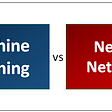## Image Processing Techniques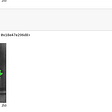## A visual way to think of macro and micro averages in classification metrics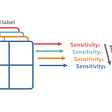## Self Driving Car in Gym Environment Using Reinforcement Learning## Making An EDA on Medical Images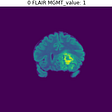## Transform video into set of images which can be used for annotation## The Overfitting …..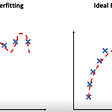## Saurabh Deoras

Software engineer and entrepreneur currently building Kubernetes infrastructure and cloud native stack for edge/IoT and ML workflows.

## [C++Algorithm] Travelling Salesman Problem Implementation in C++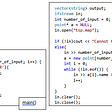## VITTY — From an Idea to a Reality## Data parallel with PyTorch on CPU’s